Select Page

## Definition

The median divides a frequency distribution into two halves. The median of set of values arranged either in ascending order or descending order of their magnitude is referred as the middle value. Median is denoted by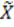( X- childa).

### Explanation

Where the number of values in a data is odd, the median shall be the middle value. And where the number of values is even, the median shall be the mean of two middle values. Once the distribution is divided into two halve by way of median then the number of values greater than the median is equal to the values smaller than the median.

#### For Example

i. The median of values; 4, 5, 6, 8, 10, 11 and 12 is 8.

ii. The median of values; 4, 6, 7, 9, 11 and 13 is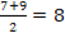From the above examples we have learned that when the number of values is odd, the median is the middle values and when the number of values is even, the median is the mean of the two middle values present in the data. In both the cases the median is the value of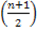th item from either ends of the data which is being arranged in ascending or descending order.

In example (i) n=7, and the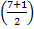th item or the 4th item from either sides is 8. And in example (ii), n=6 and the median which is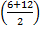th or 3.5th i.e. half way between the 3rd and 4th from either end, is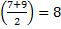.

In an array of values the position, the position of median could be determined by the following formula;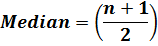## Median for Grouped Data

In case of frequency distribution the median is the value of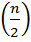th item from either end. Therefore, if we have 100 items in a frequency distribution, the median will be the value of the 50th item. In order to find the median from a frequency distribution, we need to form a separate column for cumulative frequency. The median will lie in the class which corresponds to the cumulative frequency in which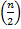lies.

The formula for median in case of frequency distribution is as follows;

Where,

l = lower class boundary of the median class, which is corresponding to the cumulative frequency in which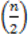lies.

h = class interval size of the median class.

f = frequency of the median class.

n = number of values, or the total frequency.

C.F = cumulative frequency of the class preceding the median class.

### For Example

The median for the following Table 14 will be;

Table 14

 Classes Frequency (f) Class Boundaries Cumulative Frequency (C.F) 0 – 9 2 -0.5 – 9.5 2 10 – 19 3 9.5 – 19.5 5 20 – 29 11 19.5 – 29.5 16 30 – 39 24 29.5 – 39.5 40 40 – 49 32 39.5 – 49.5 72 50 – 59 40 49.5 – 59.5 112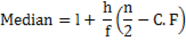Here,

n =112

i = 10

f = 32

l = 39.5

So,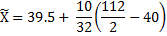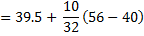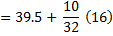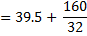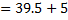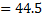Another example for calculating median by the Table 15 is provided as follows;

Table 15

 Class Marks (X) Frequency (f) Class Boundaries Cumulative Frequency (C.F) 18.5 7 13.5 – 23.5 7 28.5 12 23.5 – 33.5 19 38.5 23 33.5 – 43.5 42 48.5 35 43.5 – 53.5 77 58.5 25 53.5 – 63.5 102 68.5 8 63.5 – 73.5 110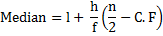Here,

n =110

h = 10

f = 35

l = 43.5

So,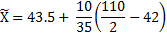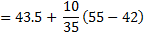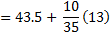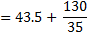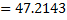Shares